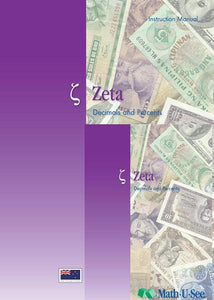or 6 weekly interest-free payments from \$10.83 withwhat's this?Demme Learning

# Math.U.See Zeta

Regular price \$65.00 \$35.88 Unit price per
Tax included. Shipping calculated at checkout.

## Math.U.See Zeta

#### Decimals, percents and other topics

Zeta extends the student’s concept of place value to the right of the decimal point. Students learn to complete core operations with decimals. The connection between fractions and decimals is presented.

Major Concepts and Skills Include:

• Expanding understanding of place value from positive powers of ten to include decimals
• Fluently adding, subtracting, multiplying, and dividing multiple digit decimals using place-value strategies
• Solving real-world problems with decimals and percentages
• Understanding the metric system and converting from one unit of measure to another

• Understanding and simplifying exponents
• Understanding negative numbers and representing them on the coordinate plane
• Using properties of operations to simplify and evaluate algebraic expressions
• Interpreting and graphing relationships between dependent and independent variables
• Understanding of plane geometry & geometric symbols
• Using ratio reasoning to solve problems

Zeta Instruction Pack includes: Instruction Manual & DVD

Zeta Student pack includes: Student Workbook & Test & Activity booklet.

Grab an extra Student Pack for an additional Student.

For a complete set to get started with Math.U.See the Zeta instruction pack & Zeta Student Pack. The Interger Block Kit & Algebra/Decimal Set are useful additions.